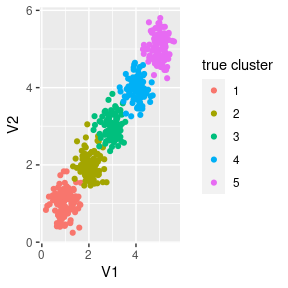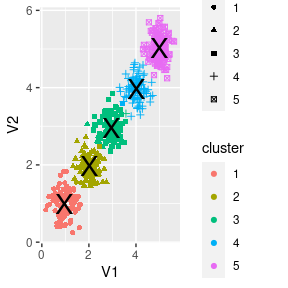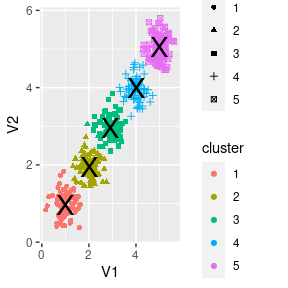# basic usage

#### 2022-08-27

Basic usage of the package.

## Basic usage

First, let’s create 5 clusters normally distributed around 1 to 5, with sd of 0.3:

data <- simulate_data(n=100, sd=0.3, nclust=5, dims=2)
data
## # A tibble: 500 × 4
##       id    V1    V2 true_clust
##    <int> <dbl> <dbl>      <int>
##  1     1 1.23  0.844          1
##  2     2 0.333 0.982          1
##  3     3 0.975 0.421          1
##  4     4 1.34  1.38           1
##  5     5 0.461 1.21           1
##  6     6 1.10  0.745          1
##  7     7 0.591 1.14           1
##  8     8 1.41  0.775          1
##  9     9 1.26  1.00           1
## 10    10 0.233 0.941          1
## # … with 490 more rows

This is how our data looks like:

data %>% ggplot(aes(x=V1, y=V2, color=factor(true_clust))) +
geom_point() +
scale_color_discrete(name='true cluster')Now we can cluster it using kmeans++:

data_for_clust <- data %>% select(id, starts_with('V'))
km <- TGL_kmeans_tidy(data_for_clust,
k=5,
metric='euclid',
verbose=TRUE)
## id column: id
## KMEans: will generate seeds
## KMeans into generate seeds
## at seed 0
## add new core from 392 to 0
## at seed 1
## done update min distance
## seed range 350 450
## picked up 55 dist was 2.30592
## add new core from 55 to 1
## at seed 2
## done update min distance
## seed range 300 400
## picked up 146 dist was 0.919783
## add new core from 146 to 2
## at seed 3
## done update min distance
## seed range 250 350
## picked up 239 dist was 0.684213
## add new core from 239 to 3
## at seed 4
## done update min distance
## seed range 200 300
## picked up 463 dist was 0.946412
## add new core from 463 to 4
## KMEans: reassign after init
## KMEans: iter 0
## KMEans: iter 1 changed 4
## KMEans: iter 1
## KMEans: iter 2 changed 3
## KMEans: iter 2
## KMEans: iter 3 changed 0

The returned list contains 3 fields:

names(km)
##  "centers" "cluster" "size"

km$centers contains a tibble with clust column and the cluster centers: km$centers
## # A tibble: 5 × 3
##   clust    V1    V2
##   <int> <dbl> <dbl>
## 1     1 4.03  3.98
## 2     2 2.02  1.97
## 3     3 4.99  5.03
## 4     4 0.971 0.985
## 5     5 2.95  2.96

clusters are numbered according to order_func (see ‘Custom cluster ordering’ section).

km$cluster contains tibble with id column with the observation id (1:n if no id column was supplied), and clust column with the observation assigned cluster: km$cluster
## # A tibble: 500 × 2
##    id    clust
##    <chr> <int>
##  1 1         4
##  2 2         4
##  3 3         4
##  4 4         4
##  5 5         4
##  6 6         4
##  7 7         4
##  8 8         4
##  9 9         4
## 10 10        4
## # … with 490 more rows

km$size contains tibble with clust column and n column with the number of points in each cluster: km$size
## # A tibble: 5 × 2
##   clust     n
##   <int> <int>
## 1     1   100
## 2     2    98
## 3     3   100
## 4     4    98
## 5     5   104

We can now check our clustering performance - fraction of observations that were classified correctly (Note that match_clusters function is internal to the package and is used only in this vignette):

d <- tglkmeans:::match_clusters(data, km, 5)
sum(d$true_clust == d$new_clust, na.rm=TRUE) / sum(!is.na(d$new_clust)) ##  0.98 And plot the results: d %>% ggplot(aes(x=V1, y=V2, color=factor(new_clust), shape=factor(true_clust))) + geom_point() + scale_color_discrete(name='cluster') + scale_shape_discrete(name='true cluster') + geom_point(data=km$centers, size=7, color='black', shape='X')## Custom cluster ordering

By default, the clusters where ordered using the following function: hclust(dist(cor(t(centers)))) - hclust of the euclidean distance of the correlation matrix of the centers.

We can supply our own function to order the clusters using reorder_func argument. The function would be applied to each center and he clusters would be ordered by the result.

km <- TGL_kmeans_tidy(data %>% select(id, starts_with('V')),
k=5,
metric='euclid',
verbose=FALSE,
reorder_func=median)
km$centers ## # A tibble: 5 × 3 ## clust V1 V2 ## <int> <dbl> <dbl> ## 1 1 0.971 0.985 ## 2 2 2.02 1.97 ## 3 3 2.95 2.96 ## 4 4 4.03 3.98 ## 5 5 4.99 5.03 ## Missing data tglkmeans can deal with missing data, as long as at least one dimension is not missing. for example: data$V1[sample(1:nrow(data), round(nrow(data)*0.2))] <- NA
data
## # A tibble: 500 × 4
##       id     V1    V2 true_clust
##    <int>  <dbl> <dbl>      <int>
##  1     1  1.23  0.844          1
##  2     2 NA     0.982          1
##  3     3  0.975 0.421          1
##  4     4  1.34  1.38           1
##  5     5  0.461 1.21           1
##  6     6 NA     0.745          1
##  7     7 NA     1.14           1
##  8     8  1.41  0.775          1
##  9     9  1.26  1.00           1
## 10    10  0.233 0.941          1
## # … with 490 more rows
km <- TGL_kmeans_tidy(data %>% select(id, starts_with('V')),
k=5,
metric='euclid',
verbose=FALSE)
d <- tglkmeans:::match_clusters(data, km, 5)
sum(d$true_clust == d$new_clust, na.rm=TRUE) / sum(!is.na(d$new_clust)) ##  0.968 and plotting the results (without the NA’s) we get: d %>% ggplot(aes(x=V1, y=V2, color=factor(new_clust), shape=factor(true_clust))) + geom_point() + scale_color_discrete(name='cluster') + scale_shape_discrete(name='true cluster') + geom_point(data=km$centers, size=7, color='black', shape='X')
## Warning: Removed 100 rows containing missing values (geom_point).## High dimensions

Let’s move to higher dimensions (and higher noise):

data <- simulate_data(n=100, sd=0.3, nclust=30, dims=300)
km <- TGL_kmeans_tidy(data %>% select(id, starts_with('V')),
k=30,
metric='euclid',
verbose=FALSE)
d <- tglkmeans:::match_clusters(data, km, 30)
sum(d$true_clust == d$new_clust, na.rm=TRUE) / sum(!is.na(d$new_clust)) ##  1 ## Comparison with R vanilla kmeans Let’s compare it to R vanilla kmeans: km_standard <- kmeans(data %>% select(starts_with('V')), 30) km_standard$clust <- tibble(id = 1:nrow(data), clust=km_standard$cluster) d <- tglkmeans:::match_clusters(data, km_standard, 30) sum(d$true_clust == d$new_clust, na.rm=TRUE) / sum(!is.na(d$new_clust))
##  0.7142857

We can see that kmeans++ clusters significantly better than R vanilla kmeans.

## Random seed

we can set the seed for the c++ random number generator, for reproducible results:

km1 <- TGL_kmeans_tidy(data %>% select(id, starts_with('V')),
k=30,
metric='euclid',
verbose=FALSE,
seed = 60427)
km2 <- TGL_kmeans_tidy(data %>% select(id, starts_with('V')),
k=30,
metric='euclid',
verbose=FALSE,
seed = 60427)
all(km1$centers[, -1] == km2$centers[, -1])
##  TRUE